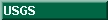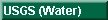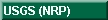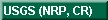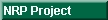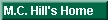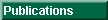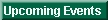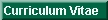### A Controlled Experiment In Ground-Water Flow Model Calibration

Mary C. Hill, Richard L. Cooley, and David W. Pollock

U.S. Geological Survey

1998, Ground Water

ABSTRACT

Nonlinear regression was introduced to ground-water modeling in the 1970's, but has been used very little to calibrate numerical models of complicated ground-water systems. Apparently, nonlinear regression is thought to be incapable of addressing such complex problems. With what we believe to be the most complicated synthetic test case used for such a study, this work investigates using nonlinear regression in ground-water model calibration.

Results of the study fall into two categories.

First, the study demonstrates how systematic use of a well designed nonlinear regression method can be used to indicate the importance of different types of data and can lead to successive improvement of models and their parameterizations. The method differs from previous methods presented in the ground-water literature in that (1) weighting is more closely related to expected data errors than is usually the case; (2) defined diagnostic statistics allow for more effective evaluation of the available data, the model, and their interaction; and (3) prior information is used more cautiously.

Second, the results challenge some commonly held beliefs about model calibration. These results indicate that (1) field measured values of hydraulic conductivity are not as directly applicable to models as their use in some geostatistical methods imply, (2) a unique model does not necessarily need to be identified to obtain accurate predictions, and (3) in the absence of obvious model bias, model error was normally distributed. The complexity of the test case involved implies that the methods used and conclusions drawn are likely to be powerful in practice.

CONCLUSIONS

In this test case, properly used nonlinear regression either produced effective, though nonunique, calibrated models capable of accurately predicting two quantities important to resource management, or provided clear evidence of model or data inadequacy. Conclusions related to demonstrating the use of the nonlinear regression method are as follows.

(1) The method of determining the weights for the weighted least-squares objective function tested in this study was used to correctly detect much smaller measurement error than would normally occur.

(2) The most conclusive indicator of model bias was unrealistic optimal parameter estimates that also had confidence intervals that excluded reasonable values. Nonrandom weighted residuals were a less conclusive indicator of model bias in the present study, but this may not always be the case, especially when models are more biased than those considered here.

(3) Including prior information in the regression diminished model accuracy when used to force optimal parameter values to be reasonable, but improved model accuracy when used to represent the hydraulic-conductivity distribution with more complexity than was supportable with the head and flow observations alone. Excluding all prior information initially allowed for clear evaluation of the contributions of different types of data.

(4) The importance of flow data was clearly demonstrated in this study. This is important because few field studies have measurements representing all of the flow leaving the system, so that problems of completely correlated parameters and the effects of a single correlation-reducing observation, as documented for the CAL0-G1 model, are probably common. These problems can be clearly characterized and understood by first representing ground-water systems very simply, and building complexity as warranted by the data and modeling objectives. Results that challenge some common practices and commonly held beliefs in model calibration include:

(5) The results of this study indicate that, given present technology, hydraulic-conductivity values measured in the field often are not as directly applicable to a numerical model of the system as would be consistent with how this data is commonly used in model calibration. Two aspects of the controlled experiment presented in this paper support this contention.

(a) In four of the models, the hydraulic-conductivity distribution was adequately represented (as evidenced by accurate simulated predictions) by an interpolation scheme in which values that were held constant were limited to the model boundaries, while nearly all values within the modeled area were estimated. Unusually accurate slug-test values were used as prior information in the regression; corresponding estimated aquifer hydraulic-conductivities differed from the slug-test values by as much as 57 percent, suggesting that direct imposition of the slug-test values, as is done in some geostatistical methods, would probably produce a less accurate model. This situation largely reflects problems of scale. Here, the numerical grid spacing was five times larger than in the true system; in field applications the scale problem is likely to be more severe.

(b) Streambed hydraulic-conductance estimates were affected by underlying subsurface heterogeneities that were not well represented by the simulated aquifer hydraulic-conductivity distribution; if field work had determined that the streambed conductance was constant along the river (which it was) and this had been imposed, the calibrated models probably would have been less accurate. This situation does not represent a scale problem as much as error in representing one part of the system affecting the parameters representing another part of the system.
(6) For at least three of the calibrated models, the fit to the regression data was nearly equally good and there was no evidence of model bias. This lack of uniqueness is probably unavoidable in complex ground-water problems, but the results of this work indicate that such nonuniqueness is not necessarily a debilitating problem. In the synthetic test case, all three models produced similar accurate predictions, probably because the data used in model construction and in the regression sufficiently constrained the solutions.

(7) Weighted residuals (observed minus simulated hydraulic heads, flows, and prior information) resulting from the regression were, in general, random and normally distributed, which is surprising because no errors had been added to the synthetically generated observations. Thus, all error was model error. It is generally thought that if model error dominates measurement error, the regression results are invalid, but the results of this work imply that the dominance of model error does not necessarily produce an inaccurate model if there is no obvious indication of model bias.

Taken with conclusion 2, conclusion 5 appears to produce a dilemma, because unrealistic parameter values are said to indicate a less accurate model, but parameter values cannot be expected to equal measured values because parameter values are accommodating model error. Yet, ranges of realistic parameter values generally are determined based on measurements. A useful resolution is derived by noting that if model error is so large that best fit parameter values are far from measured values, the resulting model is likely to produce less accurate predictions than a model for which the best-fit parameter values are close to measured values. Thus, models with more realistic best-fit parameter values are more likely to be accurate.

Conclusions 4 and 6 suggest that progress in ground-water model calibration is likely to be attained by using available data more effectively and by designing methods to collect new kinds of data, perhaps using regression methods as a guide.

mchill@usgs.gov
Last Modified: March 17, 1999